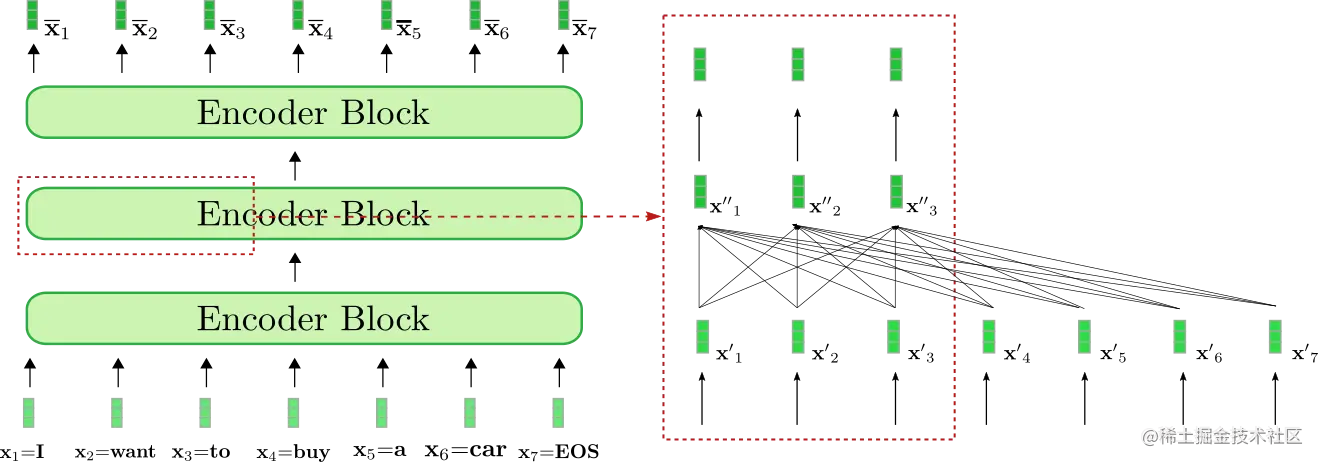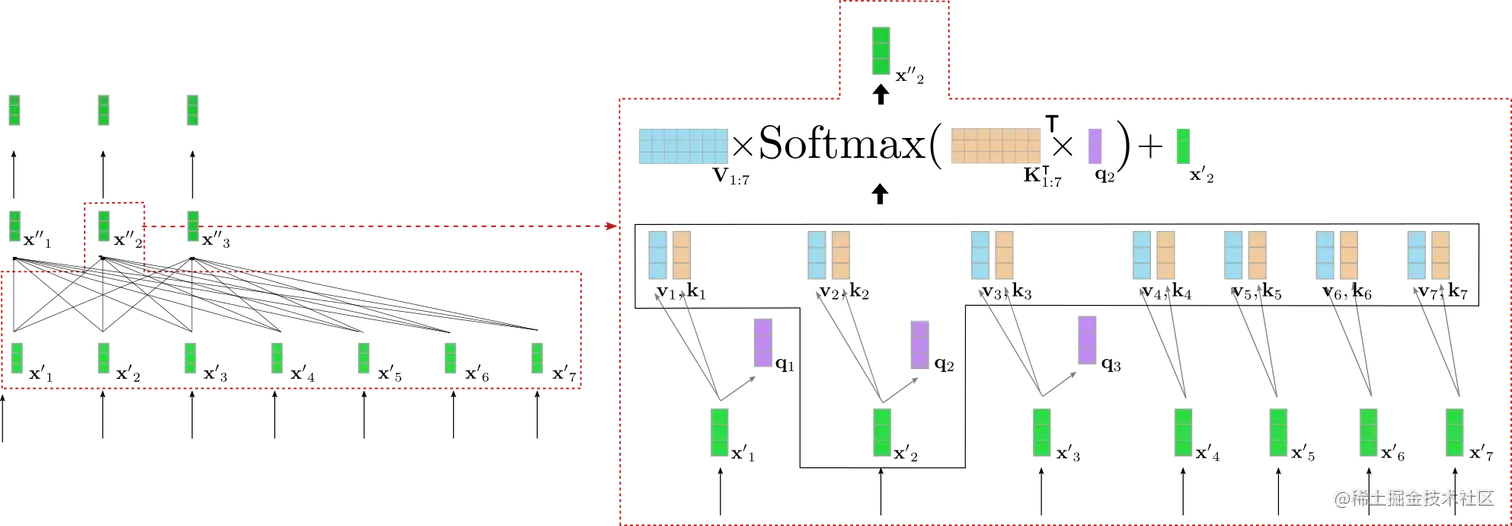# 编码器 | 基于 Transformers 的编码器-解码器模型

• 背景 - 简要回顾了神经编码器-解码器模型的历史，重点关注基于 RNN 的模型。
• 编码器-解码器 - 阐述基于 transformer 的编码器-解码器模型，并阐述如何使用该模型进行推理。
• 编码器 - 阐述模型的编码器部分。
• 解码器 - 阐述模型的解码器部分。

## 编码器

fθenc:X1:n→X‾1:nf_{\theta_{\text{enc}}}: \mathbf{X}{1:n} \to \mathbf{\overline{X}}{1:n}fθenc​​:X1:n​→X1:n​qi=Wqx′i, \mathbf{q}_i = \mathbf{W}_q \mathbf{x’}_i,qi​=Wq​x′i​, vi=Wvx′i, \mathbf{v}_i = \mathbf{W}_v \mathbf{x’}_i,vi​=Wv​x′i​, ki=Wkx′i,\mathbf{k}_i = \mathbf{W}_k \mathbf{x’}_i,ki​=Wk​x′i​, ∀i∈1,…n \forall i \in {1, \ldots n }∀i∈1,…nX′′1:n=V1:nSoftmax(Q1:n⊺K1:n)+X′1:n\mathbf{X’‘}{1:n} = \mathbf{V}{1:n} \text{Softmax}(\mathbf{Q}{1:n}^\intercal \mathbf{K}{1:n}) + \mathbf{X’}_{1:n} X′′1:n​=V1:n​Softmax(Q1:n⊺​K1:n​)+X′1:n​

a) 基于 transformer 的编码器模型如何有效地建模长程上下文表征，以及
b) 它们如何有效地处理长序列向量输入这两个方面有了比较好的理解了。

1{}^11 关于前馈层在基于 transformer 的模型中所扮演的角色的详细解释超出了本文的范畴。Yun 等人 (2017) 的工作认为前馈层对于将每个上下文向量 x′i\mathbf{x’}_ix′i​ 映射到目标输出空间至关重要，而单靠 自注意力 层无法达成这一目的。这里请注意，每个输出词元 x′\mathbf{x’}x′ 都经由相同的前馈层处理。更多详细信息，建议读者阅读论文。

2{}^22 我们无须将 EOS 附加到输入序列，虽然有工作表明，在很多情况下加入它可以提高性能。相反地，基于 transformer 的解码器必须把 BOS\text{BOS}BOS 作为第 0 个目标向量，并以之为条件预测第 1 个目标向量。

python

from transformers import MarianMTModel, MarianTokenizer
import torch

tokenizer = MarianTokenizer.from_pretrained("Helsinki-NLP/opus-mt-en-de")
model = MarianMTModel.from_pretrained("Helsinki-NLP/opus-mt-en-de")

embeddings = model.get_input_embeddings()

# create ids of encoded input vectors
input_ids = tokenizer("I want to buy a car", return_tensors="pt").input_ids

# pass input_ids to encoder
encoder_hidden_states = model.base_model.encoder(input_ids, return_dict=True).last_hidden_state

# change the input slightly and pass to encoder
input_ids_perturbed = tokenizer("I want to buy a house", return_tensors="pt").input_ids
encoder_hidden_states_perturbed = model.base_model.encoder(input_ids_perturbed, return_dict=True).last_hidden_state

# compare shape and encoding of first vector
print(f"Length of input embeddings {embeddings(input_ids).shape}. Length of encoder_hidden_states {encoder_hidden_states.shape}")

# compare values of word embedding of "I" for input_ids and perturbed input_ids
print("Is encoding for I equal to its perturbed version?: ", torch.allclose(encoder_hidden_states[0, 0], encoder_hidden_states_perturbed[0, 0], atol=1e-3))


css

    Length of input embeddings 7. Length of encoder_hidden_states 7
Is encoding for I equal to its perturbed version?: False